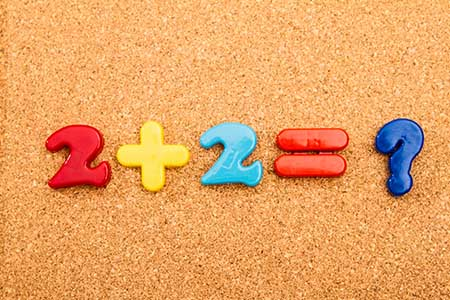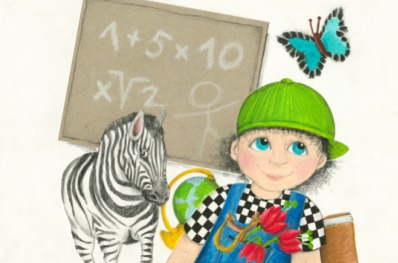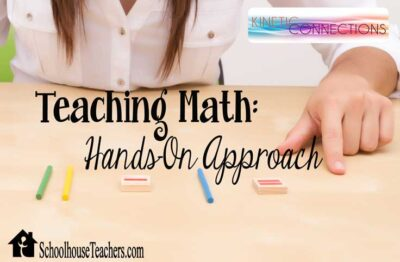# Math Center

Does your child struggle with math? Do you need to find extra practice in a particular skill? The Math Center provides easy access to many of the resources available on SchoolhouseTeachers.com.

## Featured Resources### How to Teach Elementary Math

These videos from Dr. Price demonstrate to parents various methods and strategies for teaching math to their elementary students. Worksheets for the students are provided alongside the related videos, which are presented by the grade level at which students typically learn the listed skills.

#### Kindergarten

• Rainbow Pairs to 10
• Numbers 2 Before and After
• Easy Subtraction

• Count Back Subtraction
• Difference of 1, 2, or 3
• Ten Frame Introduction and Flash Cards
• Subtracting 0 and 10
• Addition Fact Families: Counting On and Back
• Subtraction from 2-Digit Numbers Using a Written Algorithm
• Easy Multiplication 5x, 3x
• Probability: Outcomes of Familiar Events

• Subtraction Facts (Count Back 3)
• Multiplying by 10
• Introducing Fractions
• Addition and Subtraction: Doubling and Halving
• Multiplication and Division Fact Families
• Easy Division

• Multiplication and Division Review
• Equivalent Fractions
• Multiplication Tables Continued
• Division Skills Continued
• Roman Numerals to 399
• Hundreds, Tens, and Ones Place Value: Base Ten Blocks
• All Operations Review

• Division with Remainders
• Review of Multiplication and Division
• Divisibility
• Decimal Fractions: Skip Counting in Hundredths
• Comparing Fractions
• Mental Strategies: Adding “Nice” Numbers
• Factors and Multiples: Lowest Common Multiple
• Mental Strategies: Subtracting 100

• Dividing by 10, 100, and 1,000
• Adding and Subtracting Fractions with Common Denominators
• Simplifying Fractions
• Converting Between Improper Fractions and Mixed Numbers
• Converting Common Fractions into Decimals
• Doubling Large Numbers
• Calculating 1% and 0.5% of an Amount
• Review: Order of Operations
• Probability with Spinners

#### Why Teach Math and Developing Number Fluency

• Why Teach Math
• Mental Strategies
• Using Physical and Visual Models for Numbers
• Helping Children to Develop Math Thinking
• Developing “Automatic” Responses in Number Facts
• A Four-Phase Model for Teaching Operations
• More Ten Frames Examples
• Modeling Multiplication with Arrays
• Show ANY Number with a Number Line
• Combining Math and Old Technology
• Using Public Spaces to Teach Symmetry
• How to Teach Multiplication Facts
• Math and Critical Thinking
• What is REALLY Important in Math?
• Teaching Slope in the Mountains of Switzerland
• Six Simple, Free Activities Any Parent Can Use at Home to Help Their Child with Math### Everyday Games

Have you been looking for a way to add some excitement to math? One of the best ways to encourage a student to learn is by helping them have fun. Use Everyday Games to explore dozens of printable games to reinforce learning in early math concepts.

#### Math Games

• Skip Counting, Odd and Even Numbers
• Multiplication and Division
• Factors, Multiples, and Fractions
• Place Value and Decimals
• Telling Time
• Equations and Miscellaneous### Daily Math

Are you looking for some extra math practice for your students? “Daily Math” contains worksheets on a variety of math topics that you can incorporate into your regular math program to use as additional practice or as speed drills. These printable pages can be used once or multiple times as your student works on improving skills in arithmetic functions, fractions, decimals, patterns, word problems, money value, and much more.

#### Resources Available

• All Drills: Addition, Subtraction, Multiplication, and Division
• Basic Math Facts Worksheets
• Basic Skills: Place Value; Standard Measurements; Geometric Shape Identification; Ordinal Numbers; Number Patterns; Rounding Numbers; Writing Numbers as Words or Digits; Associative, Commutative, and Distributive Properties; Working with Zeroes; Order of Operations
• Intermediate Skills: Factors, Multiples, and Divisibility; Prime Numbers; Fractions and Percentages; Decimals; Subtracting Negative Numbers; Roman Numerals; Exponents; Value of Coins
• Advanced Skills: Area, Perimeter, Circumference; Angles; Finding the Mean (Average); Variables
• Miscellaneous: Adding Columns of Numbers; Solving for Rate; Comparing Ratios; Math Fun; Math Vocabulary; Probability; Describing/Defining Triangles; Inverse Operations
• Word Problems (assorted)

### World Book Online Math Games

World Book Kids includes interactive games in many subjects, including math. Follow the instructions on the web page to log in to World Book, and then follow the link to the World Book games page. Once there, select the Subject button at the top and then the Math Button.

#### Recommended Games

• Plus and Minus: Reviews addition and subtraction of one and two digits
• Times Up: Reviews multiplication facts
• Math Quest: Solve word problems using simple math facts
• Once Upon a Number: Solve word problems requiring larger numbers
• Math-a-thon: Reviews addition, subtraction, multiplication, and division facts

### SchoolhouseTeachers.com School Boxes

Would you prefer to follow a standard Scope and Sequence list of topics for grade? These virtual School Boxes have taken the work out of planning your homeschool curriculum! Each School Box is made of individual curriculum guides, one for each core subject, including Math. Each guide covers a full year of study using core courses from SchoolhouseTeachers.com.

## Hands-On MathStudents have different learning styles. More visual students may do well with pictures and white board drawings. Students who are audio learners might do better with verbal instructions. Kinetic-oriented students might do best shouting out answers to math drills while bouncing on a pogo stick. All learners, however, absorb math better with hands-on activities interspersed in their learning experience. The following courses may help inspire your creativity.

### Let’s Do Math Outside

What do bugs, nature trails, and rocks have to do with math? Let’s Do Math Outside helps elementary students learn math by counting bugs, photographing 3D shapes, discovering angles, going on a weights-and-measures scavenger hunt, and more. These printable activity instructions and worksheets are perfect for anytime families want to grab some schoolwork and head outside!

### Kinetic Connections: Teaching Math

Kinetic Connections shares practical kinesthetic learning strategies to help a parent teach a hands-on learner virtually any subject. Visit the Teaching Math articles, which come with a printable manipulative kit.

#### Resources Included

• Math Helps
• Manipulative Kit
• Memorization Helps
• Telling Time
• Reviewing with Games

### Hands-On Math Help with Cuisenaire Rods

Cuisenaire Rods are the ultimate math-learning manipulative. They are fixed shapes that represent the numbers one through ten. However, they can be made at home with connecting centimeter cubes, using designated colors for columns of one, two, three, and so on. This course uses a computer simulation program (included) to represent the rods. It teaches parents how to present the concepts. Concepts can then be taught to students using the interactive program, physical blocks (purchased separately), or handmade options.

### Special Needs

This course is a nineteen-part program to help those with children with special needs, including difficulty with math. It provides practical solutions and helpful tips for choosing curriculum and modifying and adapting instruction. For math help, see Lesson Fourteen: Assistive Technology: Struggling with Math.

#### Math Resources

On our Unit Studies and More page:

• Middle School Help: Fractions (3rd–6th)
• Middle School Help: Equations (4th–6th)
• Middle School Help: Probability and Statistics (4th–6th)
• High School Help: Finding Areas Using Reasoning (10th–12th)
• High School Help: Pythagorean Theorem (10th–12th)
• High School Help: Finding Areas Without Formulas (10th–12th)
• High School Help: Lesson on Pi (10th–12th)
• Converting Units in the Metric System (6th–8th)
• Fun with Shapes (Geometry) (4th–5th)
• How to Cross Cancel Fractions (4th-8th)
• Silly School Songs (1st–5th)
• Teach Kids Algebra (Parents)